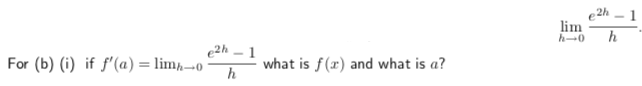# 2h-1what is f (x) and what is a?For (b) (i) if f,(a)-Inn-o-

Question
18 viewshelp_outlineImage Transcriptionclose2h-1 what is f (x) and what is a? For (b) (i) if f,(a)-Inn-o- fullscreen
check_circle

Step 1

We have to compute f(x) and value of a with the help of limit definition of derivative.

f'(a) is given below:

Step 2

Limit definition of derivative  at x=a is given  below:

Step 3

Now compare the question f\'(a) to limit definition of derivatiiv...

### Want to see the full answer?

See Solution

#### Want to see this answer and more?

Solutions are written by subject experts who are available 24/7. Questions are typically answered within 1 hour.*

See Solution
*Response times may vary by subject and question.
Tagged in

### Derivative